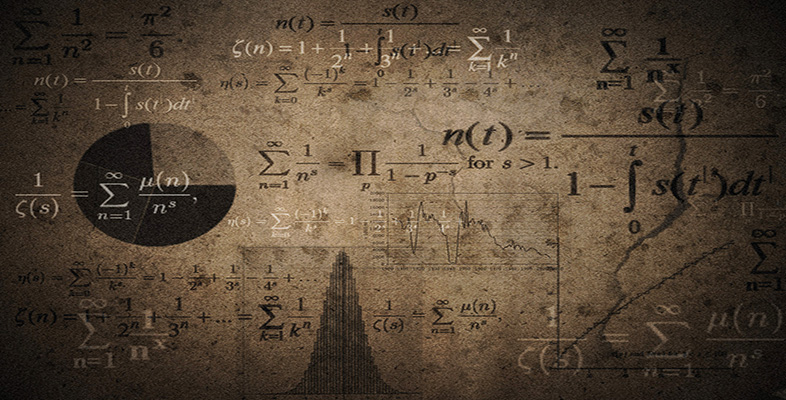Language, notation and formulas

This free course is available to start right now. Review the full course description and key learning outcomes and create an account and enrol if you want a free statement of participation.

Free course

# 2.8 Relationship symbols

There are also symbols that show the relationship between numbers or quantities. Two common ones are = and, but there are several other symbols of this type.

Symbols indicating relationships include:

• = means ‘equals’;

• ≠ means ‘does not equal’;

•means ‘is approximately equal to’;

• < means ‘is less than’;

• > means ‘is greater than’;

• ≤ means ‘is less than or equal to’;

• ≥ means ‘is greater than or equal to’.

Other symbols that are sometimes used include:

•means ‘is approximately equal to’ (an older version);

• ≡ means ‘is exactly equivalent to’;

•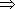means ‘implies’;

•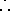means ‘therefore’;

•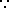means ‘because’.

Another type of symbol that you come across are abbreviations for units of measurement: for example, m for metres, kg for kilograms, and h for hours, and in imperial units ft for feet

## Example 6

What do the following mean?

• (a) time > 2s

• (b) height ≤ 100 m

• (c)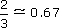• (a) The time is greater than 2 seconds.

• (b) The height is less than or equal to 100 metres.

• (c) Two-thirds is approximately equal to 0.67.

You will meet many symbols in your mathematical studies. It is important that you are sure what each one means in any given context, so that you can read it and use it appropriately.

MU120_4M6